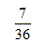Name:    Chapter 8 Post Test

1.

Select the first five terms of the sequence defined recursively. Use the pattern to write the nth term of the sequence as a function of n. (Assume that n begins with 1.)a.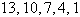b.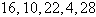c.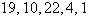d.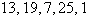e.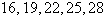2.

A deposit of \$ 1000 is made in an account that earns 6 % interest compounded monthly. The balance in the account after n months is given by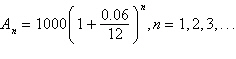Find the balance in the account after 12 years by finding the 144th term of the sequence. Round to the nearest penny.
 a. \$ 4,406,001.07 b. \$ 2040.55 c. \$ 2050.75 d. \$ 144,720.00 e. \$ 2061.00

3.

Write the first five terms of the sequence. Determine whether the sequence is arithmetic. If so, find the common difference. (Assume that n begins with 1.)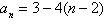a.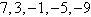Arithmetic sequence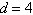b.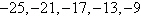Arithmetic sequence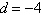c.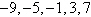Arithmetic sequenced.Arithmetic sequencee.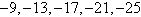Arithmetic sequence4.

Find the sum of the finite arithmetic sequence.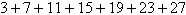a.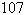b.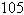c.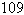d.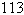e.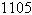5.

Find the partial sum.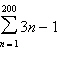a. 60,500 b. 60,702 c. 59,501 d. 60,099 e. 60,100

6.

Use a graphing utility to graph the first 10 terms of the sequence. (Assume that n begins with 1.) You may need to adjust the graphing window to the sizes indicated below to answer the question.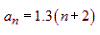a.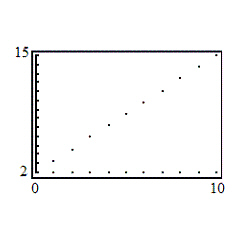d.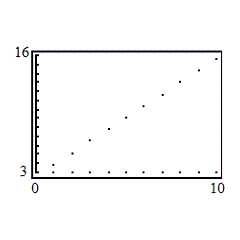b.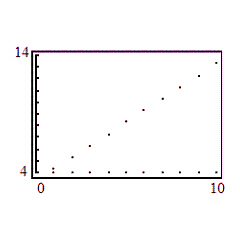e.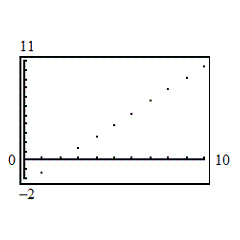c.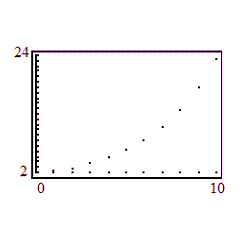7.

Find the first five terms of the geometric sequence.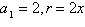a.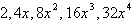b.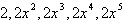c.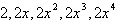d.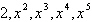e.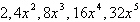8.

Find the sum of the finite geometric sequence. Round to the nearest thousandth.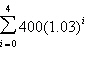a. 1673.45 b. 2123.65 c. 1723.65 d. 1886.84 e. 2187.36

9.

Find the sum using the formulas for the sums of powers of integers.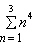a.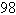b.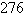c.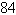d.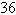e.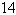10.

Use mathematical induction to solve for all positive integers n.

A factor of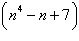is:
 a.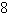b.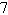c.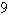d. 2 e.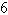11.

Calculate the binomial coefficient.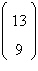a. 716 b. 715 c. 717 d. 718 e. 713

12.

Evaluate using Pascal’s Triangle.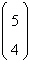a. 3 b. 6 c. 4 d. 5 e. 7

13.

Expand the binomial by using Pascal’s Triangle to determine the coefficients.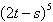a.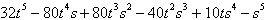b.c.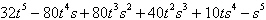d.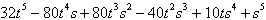e.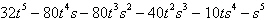14.

Find the coefficient a of the term in the expansion of the binomial.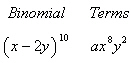a. 183 b. 179 c. 181 d. 180 e. 178

15.

Find the coefficient a of the term in the expansion of the binomial.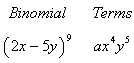a. –6,300,001 b. –6,300,002 c. –6,299,999 d. –6,300,000 e. –6,299,997

16.

Use the Binomial Theorem to expand and simplify the expression.a.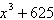b.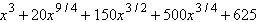c.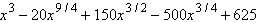d.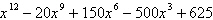e.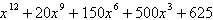17.

Evaluate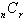using the formula from this section.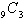a. 286 b. 504 c. 84 d. 120 e. 165

18.

Solve for n.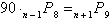a. no solution b.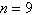c.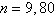d.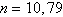e.19.

The figure shows the results of a survey in which auto racing fans listed their favorite type of racing. What is the probability that an auto racing fan selected at random lists NASCAR racing as his or her favorite type of racing?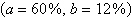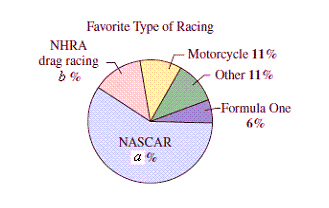a.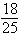b.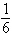c.d.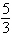e.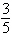20.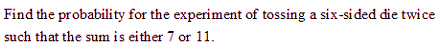a.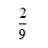b.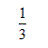c.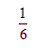d.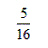e.